Mobile QR Code1. (College of Information & Communication Engineering, Sungkyunkwan University, Suwon, Korea)
2. (Department of Electronics, Pukyong National University, Busan, Korea)

Ring oscillator, dual-loop phase locked loop, frequency to voltage converter

## I. INTRODUCTION

High-performance phase-locked loop (PLL) circuits are fundamental building blocks for clock generation in many of the modern ICs. Compared with LC based PLLs, ring oscillator-based PLLs offer considerably wider tuning range and smaller area, and they efficiently produce multiple output phases. However, ring oscillator-based PLLs typically have poor phase noise and jitter performance; therefore, they are limited to low-performance applications. A wider loop bandwidth of the PLL is needed to suppress the noise of the voltage-controlled oscillator (VCO) and improve the jitter performance. However, due to the stability requirements of the PLL, the loop bandwidth is limited to approximately one-tenth of the reference frequency. Moreover, as the loop bandwidth increases, PLL performance is degraded because the reference spur is more pronounced at the PLL output. To resolve this trade-off problem, the injection locked PLL (1,2) can be a good solution. However, stability and injection timing issues continue to exist in injection-locked PLLs. To address these issues, an additional delay locked loop (DLL) (3) or a digital calibration loop (4) was added to re-time injection timing at the expense of large area and power consumption. In this paper, we solve the trade-off problems in the PLL by using a simple split-tuning technique with a frequency-to-voltage converter (FVC) and suppress the VCO noise and reference noise at the same time.

The remainder of this paper is organized as follows. In Section II, the proposed dual-loop PLL architecture is introduced. In Section III, the analysis of the proposed PLL with a FVC is presented. The measurement results are reported in Section IV, followed by the conclusions in Section V.

## II. PROPOSED ARCHITECTURE

Fig. 1 shows the architecture of the proposed dual-loop PLL. The low-bandwidth loop is composed of the conventional PLL building blocks, such as a divider, a phase and frequency detector (PFD), a charge pump (CP) and a loop filter (LF). The high-bandwidth loop utilizes only the FVC (5,6), which self-regulates the frequency of the VCO. The proposed high bandwidth path continuously converts the PLL output frequency to the control voltage ($V_{\mathrm{FVC}}$) of the VCO to detect and compensate the frequency variation of the PLL. Thus, the jitter performance of the PLL can be improved.

The FVC used in the proposed dual-loop PLL is shown in Fig. 2(a). It consists of two capacitors, $C_{\mathrm{X}}$ and $C_{\mathrm{Y}}$, a variable current source $I_{\mathrm{FVC}}$, and transistor switches. As shown in Fig. 2(a), the FVC operates through the following three steps. In the first step, the capacitor $C_{\mathrm{X}}$ is charged by the constant current $I_{\mathrm{FVC}}$ during a half period of the output clock. Then, in the second step, the accumulated charge of $C_{\mathrm{X}}$ and the charge of $C_{\mathrm{Y}}$ are equally redistributed, producing the output voltage ($V_{\mathrm{FVC}}$) that will be held by the capacitor $C_{\mathrm{Y}}$. Finally, in the third step, the capacitor $C_{\mathrm{X}}$ is discharged through the M2 switch and the next turn is prepared. To operate in this sequence, the FVC controller must generate $Φ_{\mathrm{P1}}$ and $Φ_{\mathrm{P2}}$, which are the control signals of the M1 and M2 switches. As shown in Fig. 2(b), the FVC controller uses the rising edge of $Φ_{0}$ and $Φ_{90}$ to generate $Φ_{\mathrm{P1}}$ and $Φ_{\mathrm{P2}}$. $Φ_{0}$ - $Φ_{\mathrm{0\_d }}$delay is matched to $Φ_{0}$ - $Φ_{\mathrm{P1}}$ delay, using an additional delay cell which mimics CLK-to-Q delay of the DFF. Fig. 2(c) shows the timing diagram of the FVC controller. To guarantee correct operation, the sum of the pulse widths of $Φ_{\mathrm{P1}}$ and $Φ_{\mathrm{P2}}$ should be less than one-half period of the output clock. Whenever the VCO output frequency varies, the FVC works as a compensator and suppresses the VCO noise.

Fig. 2. (a) Frequency to voltage converter circuit, (b) the FVC controller, (c) the timing diagram for the FVC controller.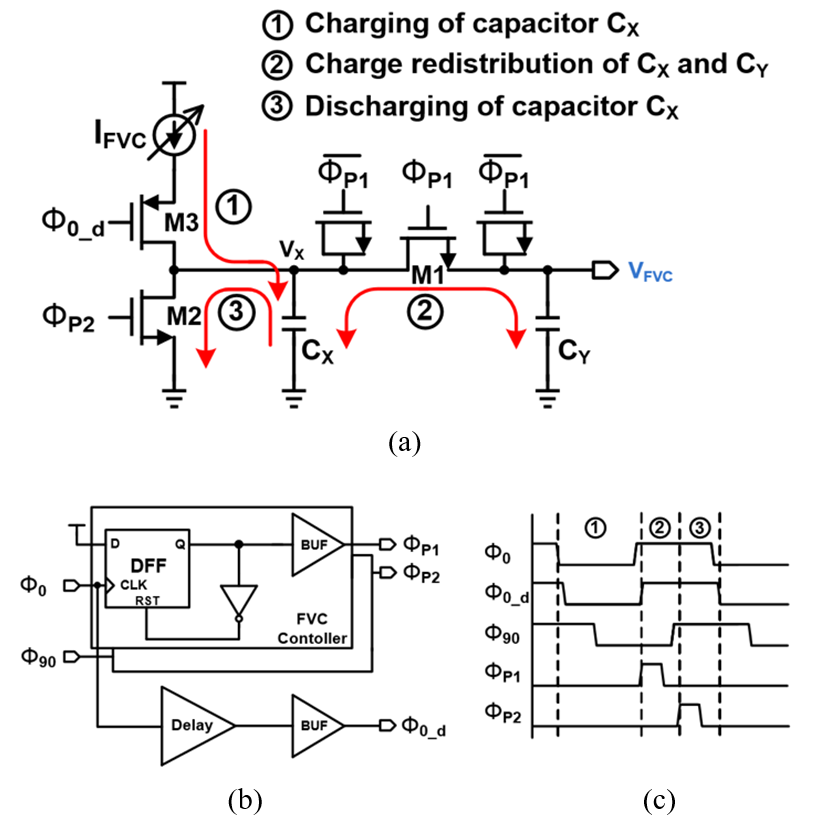Fig. 3(a) shows conceptual operating waveform of $V_{\mathrm{X}}$ and $V_{\mathrm{FVC }}$when the input frequency of the FVC is constant. As shown in Fig. 3(a), $V_{\mathrm{FVC}}$, the output voltage of the FVC, becomes close to $V_{\mathrm{X,MAX}}$ after several iterations of charge sharing between $C_{\mathrm{X}}$ and $C_{\mathrm{Y}}$. That is, if the input frequency is constant, the output voltage of the FVC can be expressed by the following equation:

Fig. 3. (a) Conceptual operating waveform, (b) the simulation results of of $V_{\mathrm{X}}$ and $V_{\mathrm{FVC}}$.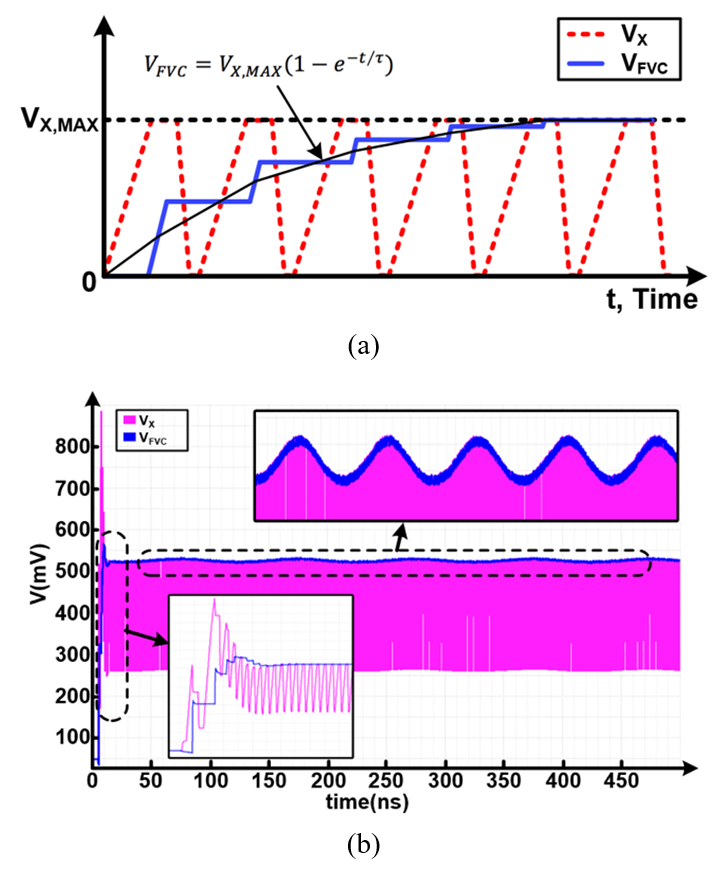##### (1)
$V_{FVC}=V_{X,MAX}\left[1- \left(\frac{C_{X}}{C_{X}+C_{Y}}\right)^{N}\right],$

where N is the number of cycles. Using Eq. (1), the absolute error $ΔV_{\mathrm{FVC}}$, the difference between $V_{\mathrm{X,MAX}}$ and $V_{\mathrm{FVC}}$ can be expressed by

##### (2)
$ΔV_{FVC}=\left| V_{X,MAX}- V_{FVC}\right| =V_{X,MAX}\left(\frac{C_{X}}{C_{X}+C_{Y}}\right)^{N}$

If $C_{\mathrm{X}}$ and $C_{\mathrm{Y}}$ are equal, $ΔV_{\mathrm{FVC}}$ becomes less then 1% of $V_{\mathrm{X,MAX}}$ when N = 7. Therefore, we can conclude that $V_{\mathrm{FVC}}$ quickly tracks $V_{\mathrm{X,MAX}}$ with negligible delay (with a small N value). Because N is the quotient of $\frac{t}{1/f_{OUT}}$, we can approximate Eq. (1) to the following equation of a continuous form.

##### (3)
$V_{FVC}=V_{X,~ MAX}\left[1- \left(\frac{C_{X}}{C_{X}+C_{Y}}\right)^{t{f_{OUT}}}\right]$

where $f_{\mathrm{OUT}}$ is the frequency of the VCO output, which is the input of the FVC. From Eq. (3), the $V_{\mathrm{FVC}}$ can be expressed as an exponential function:

##### (4)
$V_{FVC}=V_{X,~ MAX}\left(1- e^{- t/\tau }\right)$

##### (5)
$\tau =\left| \frac{1}{\log \left(\frac{C_{X}}{C_{X}+C_{Y}}\right)f_{OUT}}\right|$

Finally, the transfer function of the FVC circuit can be expressed as follows.

##### (6)
$H_{FVC}\left(s\right)=\frac{K_{FVC}}{1+s/\omega _{p}}$

##### (7)
$\omega _{p}=\left| \log \left(\frac{C_{X}}{C_{X}+C_{Y}}\right)f_{OUT}\right|$

where $K_{\mathrm{FVC}}$ is the dc gain from the input frequency $f_{\mathrm{OUT}}$ to the output voltage $V_{\mathrm{FVC}}$.

Fig. 3(b) shows the simulation results of $V_{\mathrm{X}}$ and $V_{\mathrm{FVC}}$ when the input frequency varies between 1.48 GHz and 1.52 GHz. The changes in the input frequency induce $V_{\mathrm{X,MAX}}$’s change, and $V_{\mathrm{FVC}}$ quickly follows $V_{\mathrm{X,MAX}}$ as predicted by Eq. (2). Because delay between $V_{\mathrm{FVC}}$ and $V_{\mathrm{X,MAX}}$ is negligible, the gain from the input frequency to $V_{\mathrm{FVC}}$ can be modified to the gain from the input frequency to $V_{\mathrm{X,MAX}}$ as follows:

##### (8)
$K_{FVC}=\frac{\partial V_{FVC}}{\partial f_{OUT}}=\frac{\partial V_{X,MAX}}{\partial f_{OUT}}\\ ~ ~ ~ ~ ~ ~ ~ ~ ~ ~ =\frac{\partial }{\partial f_{OUT}}\left(\frac{I_{FVC}}{2C_{X}f_{OUT}}\right)\\ ~ =- \frac{I_{FVC}}{2C_{X}\left(f_{OUT}\right)^{2}}~ ,$

The dimension of the $K_{\mathrm{FVC}}$ is V/Hz. The $K_{\mathrm{FVC}}$ in the actual design is 0.37nV/Hz. The bandwidth of the FVC, $\omega _{P}$, is estimated as 450 MHz when $I_{\mathrm{FVC}}$, $C_{\mathrm{X}}$, $C_{\mathrm{Y}}$, $f_{\mathrm{OUT}}$ are 500 ${\mathrm{\mu}}$A, 300 fF, 300 fF, 1.5 GHz, respectively.

## III. ANALYSIS OF THE PROPOSED PLL

Fig. 4 shows the linear model of the proposed dual-loop PLL with the effect of reference noise and VCO noise. It consists of low-bandwidth and high-bandwidth paths. First, the transfer function of the loop filter, Z(s), is given as

##### (9)
$Z\left(s\right)=\frac{RC_{1}s+1}{s\left(RC_{1}C_{2}s+C_{1}+C_{2}\right)}~ ,$

where R and $C_{1}$, $C_{2}$ are the resistor and capacitors of the loop filter, respectively. Without noise sources, the open-loop transfer function of the proposed dual-loop PLL is expressed as

##### (10)
$H_{open}\left(s\right)=\frac{\frac{I_{CP}}{2\pi }Z\left(s\right)\frac{K_{VCO1}}{s}\frac{1}{N}}{1+\frac{1}{M}H_{FVC}\left(s\right)K_{VCO2}}~ ,$

where M and N are the frequency dividing ratio in the high-bandwidth path and low-bandwidth path. In this PLL design, M and N are equal to 1 and 32, respectively. $K_{\mathrm{VCO1}}$ and $K_{\mathrm{VCO2}}$ are the VCO gains of the low bandwidth path and the high bandwidth path, respectively.

Fig. 4. Linear model of the proposed dual-loop PLL with input noise and VCO noise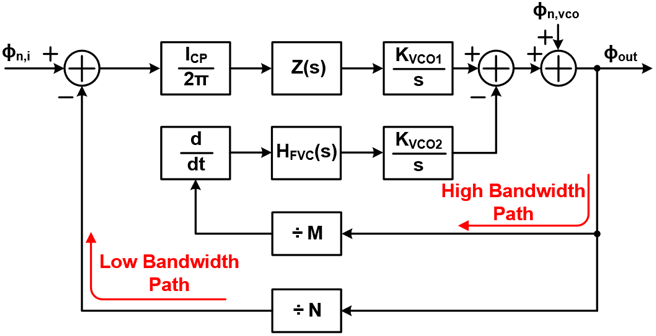Fig. 5 shows the magnitude and phase responses of the proposed dual-loop PLL. Since the pole formed by the high-bandwidth path is located at a high frequency, the loop stability of the proposed PLL is not affected by the FVC circuit.

Fig. 5. Magnitude and phase responses of the proposed dual-loop PLL.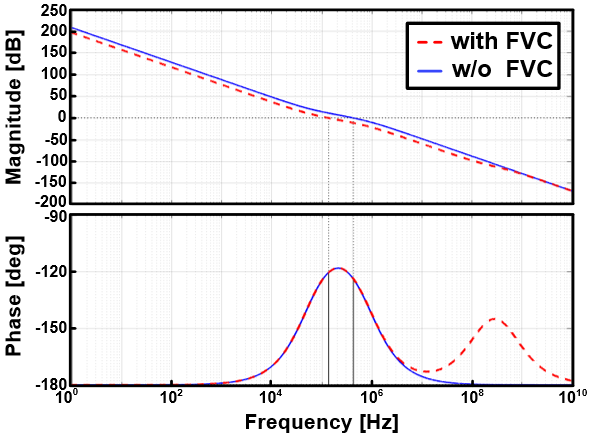To formulate the PLL output phase noise due to the input phase noise, we derive the transfer function from the input phase ($Φ_{\mathrm{n,i}}$) to the PLL output phase ($Φ_{\mathrm{out}}$) using the linear phase model of Fig. 4 while setting the excess phase of the VCO ($Φ_{\mathrm{n,vco}}$) to zero.

##### (11)
$\frac{\phi _{out}}{\phi _{n,i}}=\frac{\frac{I_{CP}}{2\pi }\frac{K_{VCO1}}{s}Z\left(s\right)}{1+\frac{1}{M}H_{FVC}\left(s\right)K_{VCO2}+\frac{1}{N}\frac{I_{CP}}{2\pi }\frac{K_{VCO1}}{s}Z\left(s\right)}$

Also, to formulate the PLL output phase noise due to the VCO phase noise, we derived the transfer function from the VCO phase ($Φ_{\mathrm{n,vco}}$) to the PLL output phase ($Φ_{\mathrm{out}}$). In this derivation, we also used the linear phase model of Fig. 4 and set the excess phase of the input ($Φ_{\mathrm{n,i}}$) to zero to model a clean reference.

##### (12)
$\frac{\phi_{\text {out}}}{\phi_{\text {n,vco}}}=\frac{1}{1+\frac{1}{M} H_{F V C}(s) K_{V C O 2}+\frac{1}{N} \frac{I_{C P}}{2 \pi} \frac{K_{V C O 1}}{s} Z(s)}$

As expressed in the above equation, unlike the conventional PLL, the “$H_{\mathrm{FVC}}$(s)$K_{\mathrm{VCO2}}$/M” term is generated in the denominator of the noise transfer functions due to the high-bandwidth loop. Thus, it is possible to reduce the VCO noise and reference noise at the same time.

Fig. 6 shows the calculated results of the noise transfer functions. The dotted lines represent the results obtained for the conventional PLL without the FVC circuit and the solid lines represent the results for the proposed dual-loop PLL with the FVC circuit. Because the loop bandwidth of the conventional PLL is set to 1 MHz, the noise reduction in the proposed dual-loop PLL is noticeable near 1 MHz. In other words, the proposed dual-loop PLL with the high-bandwidth path can suppress the VCO noise and reference noise as described in the Eqs. Eq. (11, 12).

Fig. 6. The calculated noise transfer functions of the PLL with and without FVC.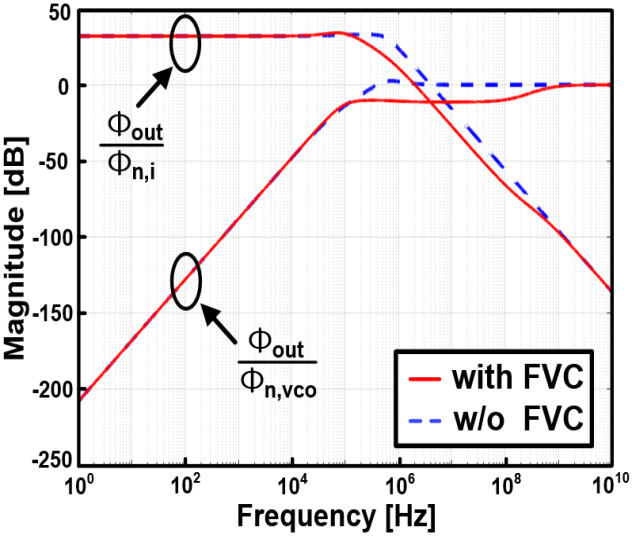Fig. 7(a) shows the transfer function of the input noise, Eq. (11), as a function of the high-bandwidth path gain. As the gain increases, the input noise of the proposed is further suppressed by the high-bandwidth path. In Fig. 7(b), Eq. (12), the noise transfer function for the VCO, is plotted. As the gain increases, the -3-dB bandwidth of the dual-loop PLL increases, and the VCO noise is further suppressed.

Fig. 7. Transfer functions of the (a) input noise, (b) VCO noise.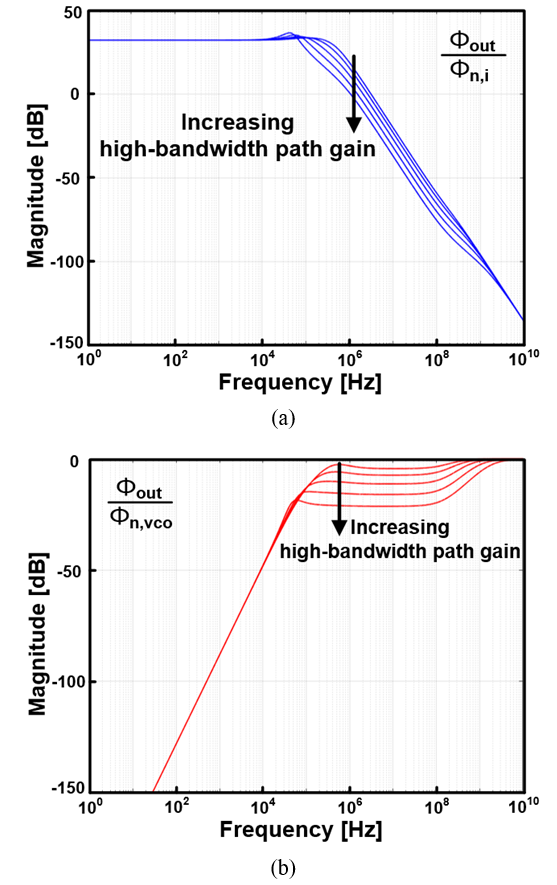Fig. 8 shows the simulation results of the output clock when 10-MHz triangular noise is injected into the VCO power supply, VDD\_REG. The frequency variation of the output clock is 37.5 MHz without the FVC and 7.6 MHz with the FVC. The $V_{\mathrm{FVC}}$ moves even after the PLL is locked to compensate the frequency variation due to the noise injection. The simulation results show that the high bandwidth path can reduce the frequency variation by 80 %.

Fig. 8. Simulated frequency variation of the output clock with supply noise injection.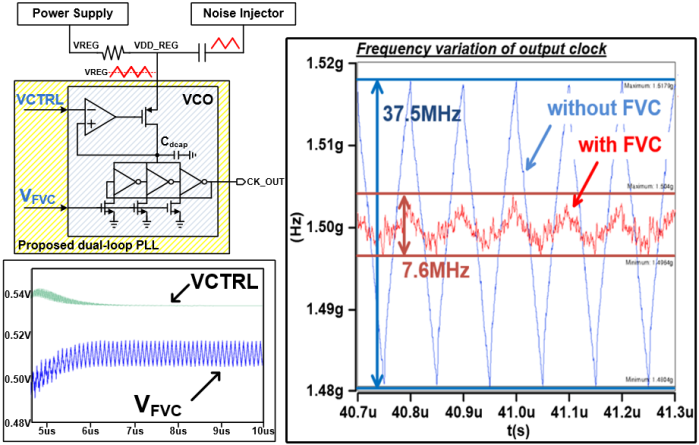In summary, the out-band phase noise of the dual-loop PLL arising from the VCO noise will be suppressed by the high bandwidth path and the in-band phase noise arising from the input noise will also be suppressed by the split-tuning technique with FVC.

## IV. MEASUREMENT RESULTS

The proposed dual-loop PLL was implemented in a 28-nm CMOS process and the operation frequency was 1.5 GHz. The proposed PLL occupies 350 μm ${\times}$ 650 μm, and consumes 4 mW from a 1.0 V supply while operating at 1.5 GHz. The high-bandwidth path occupies 70 μm ${\times}$ 50 μm, and consumes 600 μW that is 15% of the total power. We measured the phase noise of the PLL outputs when 10-MHz sinusoidal noise was injected into the VCO. The results are shown in Fig. 9. With noise injection, the phase noise of the free-running VCO at 1.5~GHz was -69 dBc/Hz at a 1-MHz offset with power consumption of 2 mW.

Fig. 9 shows the measured phase noise spectra of 1.5~GHz output clocks with and without the FVC. Without the FVC, the conventional PLL achieves the phase noise of -78.4 dBc/Hz at a 1-MHz offset. With the FVC, the phase noise was reduced to -88.6 dBc/Hz at a 1-MHz offset. Moreover, the phase noise of the proposed PLL with the FVC was reduced in the offset frequency range from 10 kHz to 1 MHz in comparison with the PLL without the FVC. In addition, the out-band spurs were also eliminated in the proposed dual-loop PLL.

Fig. 9. Measured phase noise of the 1.5-GHz PLL outputs with and without FVC.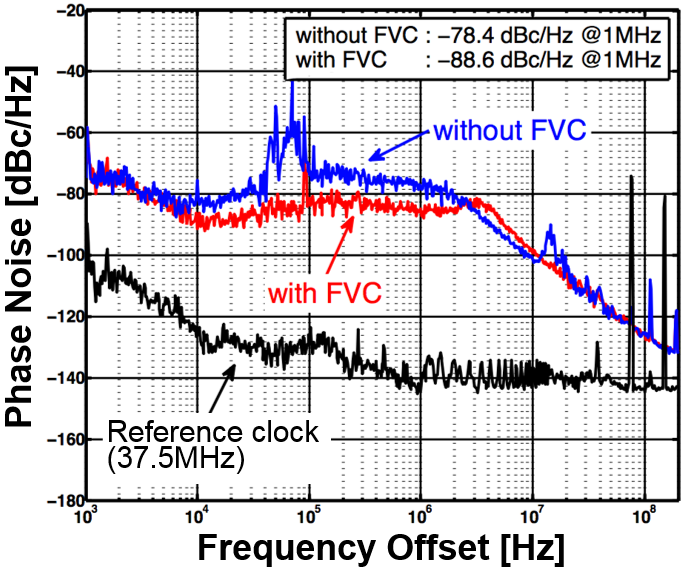Table 1. Performance summary

 Process 28 nm CMOS Oscillator Type Ring Output Frequency 1.5 GHz Reference Frequency 37.5 MHz *PN @1-MHz (without FVC) -78.4 dBc/Hz *PN @1-MHz (with FVC) -88.6 dBc/Hz *Reference Spur (without FVC) -38.70 dBc *Reference Spur (with FVC) -59.33 dBc Chip Area 0.23 mm2 Power 4 mW

*with the power supply noise injection

Fig. 10 presents the PLL output spectrum within the frequency range of 1 MHz from the carrier frequency. By turning on the FVC, we could reduce the noise level by 10dB. Fig. 11 shows the high-frequency spurs located at $f_{\mathrm{ref}}$, 2${\times}$$f_{\mathrm{ref}}, 3{\times}$$f_{\mathrm{ref}}$. The proposed PLL effectively reduced the reference spurs. For the 3rd reference spur at 3${\times}$$f_{\mathrm{ref}}$, the proposed dual-loop PLL achieved a -59.33 dBc spur level, while the conventional PLL had a -38.70 dBc spur level. The measurement results demonstrate that the proposed dual-loop PLL can suppress both the VCO noise and reference noise simultaneously. A die photo is shown in Fig. 12, and Table 1 summarizes the performance of the test chip.

Fig. 10. Measured output spectrums of the PLL within the frequency range of 1-MHz from the carrier frequency.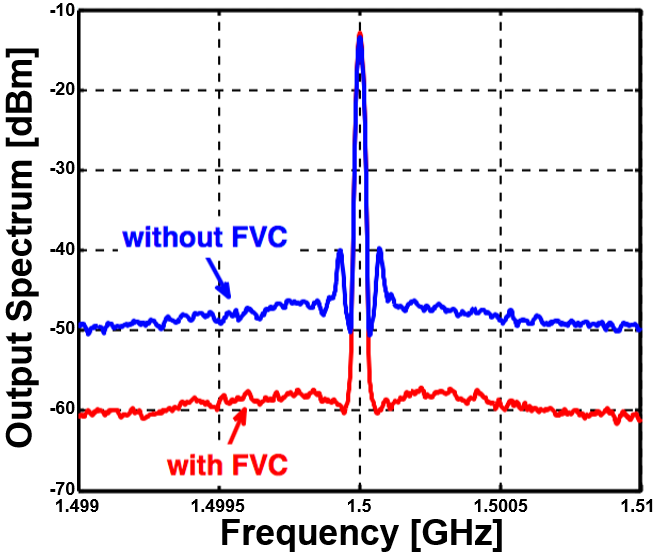Fig. 11. Measured spectrum of the PLL output (a) without FVC, (b) with FVC.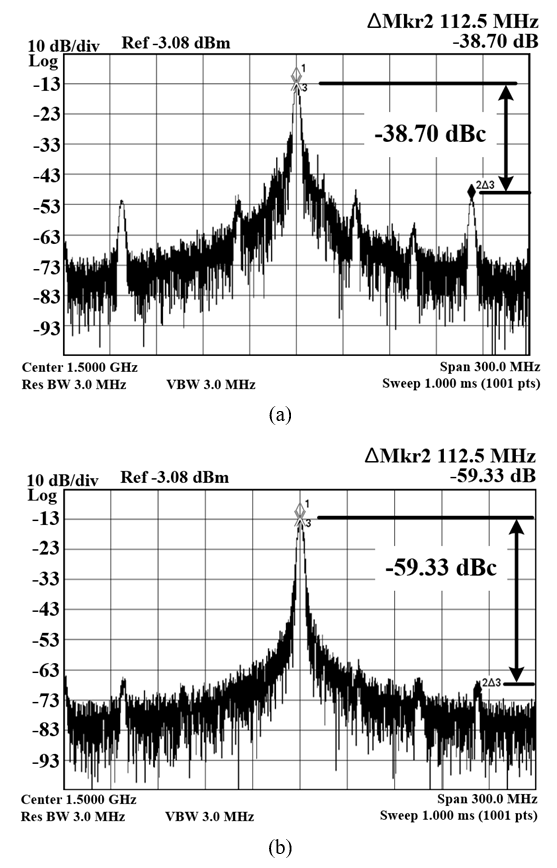## V. CONCLUSIONS

In this paper, a dual-loop PLL with an FVC was proposed, and its implementation was described. By employing the FVC, the PLL forms a high-bandwidth path that suppresses the noise of the VCO and the reference clock. In the proposed architecture, the PLL bandwidth can be maximized to suppress the VCO phase noise without the need for complex circuit components. The proposed dual-loop PLL can be used either as a low-jitter clock generator in digital systems or as a frequency synthesizer in wireless communication systems.

### ACKNOWLEDGMENTS

This work was supported in part by the Basic Science Research Program through the National Research Foundation of Korea under Grant 2016R1D1A1B0 4933413 and in part by the program for fostering next-generation researchers in engineering of National Research Foundation of Korea (NRF) funded by the Ministry of Science, ICT & Future Planning (2017H1D8A2031628).

### REFERENCES

1
Lee Jri, Wang Huaide, May 2009, Study of Subharmonically Injection-Locked PLLs, IEEE Journal of Solid-State Circuits, Vol. 44, No. 5, pp. 1539-15532
Ye Sheng, Jansson Lars, Galton Ian, Dec 2002, A Multiple-Crystal Interface PLL With VCO Realignment to Reduce Phase Noise, IEEE Journal of Solid-State Circuits, Vol. 37, No. 12, pp. 1795-18303
Huang Yi-Chieh, Liu Shen-Iuan, Feb 2012, A 2.4GHz sub-harmonically injection-locked PLL with self-calibrated injection timing, in Solid-State Circuits Conference Digest of Technical Papers (ISSCC), pp. 338-3404
Grollitsch Werner, Nonis Roberto, Sept 2016, A fractional-N, all-digital injetion-locked PLL with wide tuning range digitally controlled ring oscillator and Bang-Bang phase detection for temperature tracking in 40nm CMOS, in European Solid-State Circuits Conference (ESSCIRC), pp. 201-2045
Choi Young-Shig, Sept 2013, A Negative Feedback Looped Voltage-Controlled Ring Oscillator With Frequency Voltage Converter, IEEE Transactions on Microwave Theory and Techniques, Vol. 61, No. 9, pp. 3271-32766
Bui Hung Tien, Savaria Yvon, April 2008, Design of a High-Speed Differential Frequency-to-Voltage Converter and Its Application in a 5-GHz Frequency-Locked Loop, IEEE Transactions on Circuits and Systems I, Vol. 55, No. 3, pp. 766-774## Author

Xuefan Jin received the B.S. degree from the Department of Electronic Communication Engineering, Yanbian University of Science and Techno-logy, Yanji, China, in 2010. He is currently pursuing the combined master’s/Ph.D. degree in electrical engineering from Sungkyunkwan University, Suwon, South Korea. His current research interests include high-speed serial links, phase-locked loops, and clock generation circuit designs.

Kee-Won Kwon received his B.S. degree in metallurgical engineering from Seoul National University, in 1988. He also received his M.S. degree in electrical engineering and the Ph.D. degree in materials science and engineering from Stanford University, Stanford, CA, in 2000 and 2001, respectively. From 1990 to 1995, he was with Samsung Electronics, Giheung, Korea, where he developed tantalum pentoxide dielectric thin films and successfully implemented them into the commercial product of DRAM. In 2000, he worked for Maxim Integrated Products, Sunnyvale, CA where he was involved in two projects of data converting circuit design. He rejoined Samsung Electronics in 2001, and worked in the areas of high performance DRAM designs including Rambus DRAM and XDR DRAM. In 2007, he moved to Sungkyunkwan University, where he is doing research on memory IP design, and low power high speed circuit solutions for analog and mixed-signal devices.

Young-Shig Choi received B.S. degree in Electronics Engineering from Kyungpook National University in 1982, M.S. degree from Texas A & M University in 1986 and Ph.D. from Arizona State University in Electrical Engineering in 1993. From 1987 to 1999, he was with Hyundai Electronics (Now SK Hynix) as a principal circuit design engineer where he has been involved in the development of communication and mixed signal chips. In March 2003, he joined the faculty of Dept. of Electronics, Pukyong National, where he is currently a Professor. His current interests include PLL and DLL design.

Jung-Hoon Chun received the B.S. and M.S. degrees in electrical engineering from Seoul National University, Seoul, South Korea, in 1998 and 2000, respectively, and the Ph.D. degree in electrical engi-neering from Stanford University, Stanford, CA, USA, in 2006. From 2000 to 2001, he was with Samsung Electronics, Hwaseong, South Korea, where he developed BiCMOS RF front-end IC for wireless communication. From 2006 to 2008, he was with Rambus Inc., Los Altos, CA, USA, where he worked on high-speed serial interfaces such as FlexIO, XDR, and XDR2. He is currently an Associate Professor with Sungkyunkwan University, Suwon, South Korea. He also consults for the several IC design and foundry companies in South Korea and Silicon Valley. His current research interests include high- speed serial links, image sensors, new memory devices, power management ICs, on-chip electrostatic discharge protection, and I/O design. Dr. Chun was a recipient of the IEEE SOI Conference Best Paper Award in 2010, the IEEE CICC Best Paper Award in 2008, the Benhamou SGF Fellowship from 2003 to 2005, and the Gold Medal at the Humantech Thesis Competition in 1998. He served on the Technical Program Committee of the IEEE A-SSCC from 2009 to 2011 and from 2014 to 2017.